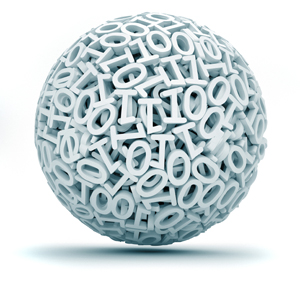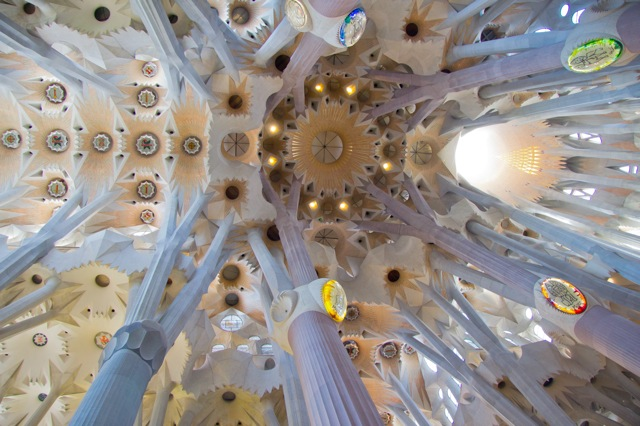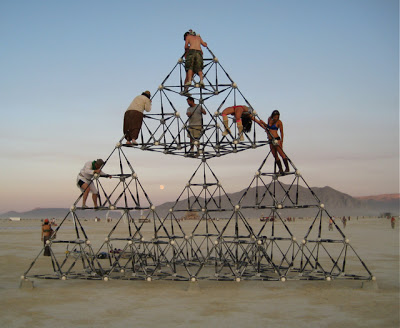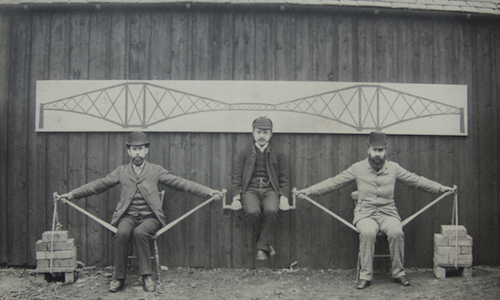## Plus Blog

August 7, 2014Most people are aware of the fact that computers work using strings of 0s and 1s. But how do you write numbers using only those two symbols?

To see how, let's first remind ourselves of how the ordinary decimal way of writing numbers works. Let's take the number 4302 as an example. The digit 4 in this number doesn't stand for the number 4, rather it stands for 4000, or 4 x 1000. Similarly, 3 doesn't stand for 3 but for 300 = 3 x 100, 0 stands for 0 x 10, and 2 stands for 2 x 1. So 4302 means

4 x 1000 + 3 x 1000 + 0 x 10 + 2 x 1.

Similarly, 7396 stands for

7 x 1000 + 3 x 100 + 9 x 10 + 6 x 1.

What do the numbers 1000, 100, 10 and 1, which appear in these expressions, have in common? They are all powers of 10:

1000 = 103
100 = 102
10 = 101
1 = 100.

To write a number in decimal notation, you first write it as a sum of consecutive powers of 10 (with the largest power on the left) and then pull out the coefficients of these powers. We can do the same with powers of 2 rather than 10. For example, the binary number 110 stands for

1 x 22 + 1 x 21 + 0 x 20 = 4 + 2 +0 = 6 (written in decimal).

And the binary number 10001 stands for

1 x 24 + 0 x 23 + 0 x 22 + 0 x 21 + 1 x 20 = 16 + 0 + 0 + 0 + 1 = 17 (written in decimal).

You can convince yourself that a binary number only consists of the digits 0 or 1: when you write a number as a sum of consecutive powers of 2, no other coefficients are necessary.

This sorts out the natural numbers, but what about numbers that have a fractional part? To write a number between 0 and 1 in binary, you play the same game using powers of 1/2 instead of powers of 2. For example,

0.75 = 1/2 + 1/4 = 1 x 1/21+ 1 x 1/22.

In binary, 0.75 is written as 0.11. The binary number 0.1001 stands for the decimal number

1 x 1/21+ 0 x 1/22 + 0 x 1/23+ 1 x 1/24 = 1/2 + 1/16 =0.5625.

Easy!

You can find out more about the positional way of writing numbers here and about the use of 0s and 1s in logical operations here.

July 31, 2014This beautiful image shows details of the ceiling of the Sagrada Familia basilica in Barcelona, illustrating architect Antoni Gaudí's love of mathematical design. © Tim Jones.

The picture is one of the images that appear in the book 50 visions of mathematics, which celebrates the 50th anniversary of the Institute of Mathematics and its Applications.

You can find out more about maths and architecture here and about maths and art here.

July 30, 2014

A perfect number is a natural number whose divisors add up to the number itself. The number 6 is a perfect example: the divisors of 6 are 1, 2 and 3 (we exclude 6 itself, that is, we only consider proper divisors) and

1+2+3 = 6.If a non-perfect number were an animal, it might look something like this.

Hooray! People have known about perfect numbers for millennia and have always been fascinated by them. Saint Augustine (354–430) thought that the perfection of the number 6 is the reason why god chose to create the world in 6 days, taking a rest on the 7th. The Greek Nicomachus of Gerasa (60-120) thought that perfect numbers produce virtue, just measure, propriety and beauty. Numbers that are not perfect, for example numbers whose proper divisors add up to more than the number itself, Nichomachus found very disturbing. He accused them of producing excess, superfluity, exaggerations and abuse, and of being like animals with "ten mouths, or nine lips, and provided with three lines of teeth; or with a hundred arms, or having too many fingers on one of its hands."

If you play around with numbers for a while you will see why people have always been so fond of perfect numbers: they are very rare. The next one after 6 is 28, then it's 496, and for the fourth perfect number we have to go all the way up to 8128. Throughout antiquity, and until well into the middle ages, those four were the only perfect numbers that were known. Today we still only know of 51 of them, even though there are fast computers to help us find them. The largest so far, discovered in December 2018, has over 49 million digits.

Will we ever find another one? We can't be sure — mathematicians believe that there are infinitely many perfect numbers, so the supply will never run out, but nobody has been able to prove this. It's one of the great mysteries of mathematics. You can find out more in Number mysteries.

July 24, 2014

In the process of writing an article on curvature we got entirely distracted by making a geogebra worksheet showing the tangent, normal and osculating circle to any smooth function. The meaning and mathematics of all these terms is revealed in this article, but if you fancy getting your hands dirty yourself, have a play with the worksheet below. Please post your favourite function as a comment – the curvier and wigglier the better!

You can use this geogebra worksheet to see the tangent, normal and osculating circle of any smooth curve you choose - just change the equation f(x) in the left-hand panel.

July 24, 2014Bat country. Image designed by Gwen Fisher, engineered by Paul Brown. © Gwen Fisher.

These lucky people are climbing around a 22 feet (6.7 metres) tall structure composed of 384 softball bats, 130 soft balls and a couple of thousand pounds of steel. The structure represents a Sierpinski tetrahedron: a fractal which has finite volume but infinite area. The image only shows an approximation of the fractal of course, as it would be impossible to make a full-on Sierpinski tetrahedon with its infinite intricacy, but it's beautiful anyway!

The picture, designed by Gwen Fisher and engineered by Paul Brown, is one of the images that appears in the book 50 visions of mathematics, which celebrates the 50th anniversary of the Institute of Mathematics and its Applications.

You can find out more about fractals here.